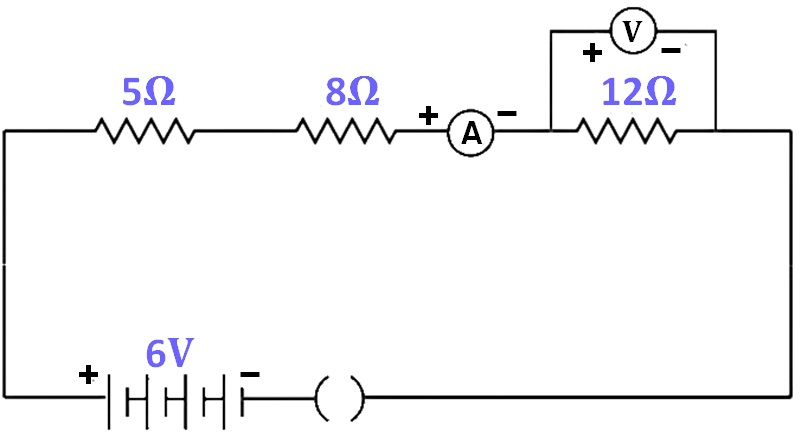Past Year - 3 Mark Questions

Class 10
Chapter 12 Class 10 - Electricity

## Consider the following circuit:## What would be the readings of the ammeter and the voltmeter when key is closed? Give reason to justify your answer.

Total resistance, R = R 1 + R 2 + R 3

= 5 + 8 + 12

= 25 ohm

V = 6 volts

According to ohm’s law,

V = IR

I = V / R

= 6 / 25

= 0.24 A

Current through 12 ohm resistor = 0.24 A

Voltage = I * R

= 0.24 * 12

= 2.88 V

Therefore,

• Ammeter reading = 0.24 A
• Voltmeter reading = 2.88 V

Learn in your speed, with individual attention - Teachoo Maths 1-on-1 Class